# Pool

The swimming pool is 10 m wide and 8 m long and 153 cm deep. How many hectoliters of water is in it, if the water is 30 cm below its upper edge?

Correct result:

V =  984 hl

#### Solution: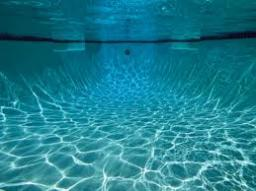Our examples were largely sent or created by pupils and students themselves. Therefore, we would be pleased if you could send us any errors you found, spelling mistakes, or rephasing the example. Thank you!

Leave us a comment of this math problem and its solution (i.e. if it is still somewhat unclear...):Math student
IT'S 9 CM NOT 10 CM. SO IT SHOULD BE 4004.Dr Math
corrected, thank youTips to related online calculators
Do you want to convert length units?
Do you know the volume and unit volume, and want to convert volume units?

## Next similar math problems:

• Telegraph poles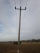The bases of two adjacent telegraph poles have a height difference of 10.5 m. How long do the wires connect the two poles if the slope is 39° 30´?
• AL wireWhat is the weight of an aluminum wire 250 m long with a diameter of 2 mm, if the density of aluminum is p = 2700 kg/m cubic. Determine to the nearest gram.
• The water tankThe water tank has the shape of a sphere with a radius of 2 m. How many liters of water will fit in the tank? How many kilograms of paint do we need to paint the tank, if we paint with 1 kg of paint 10 m2?
• Runcated pyramid teapot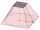The 35 cm high teapot has the shape of a truncated pyramid with the length of the edge of the lower square base a=50 cm and with the edges of the rectangular base b: 20 cm and c: 30 cm. How many liters of water will fit in the teapot?
• Alcohol from potatoes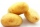In the distillery, 10 hl of alcohol can make from 8 t of potatoes. The rectangular field with dimensions of 600 m and 200 m had a yield of 20 t of potatoes per hectare. How many square meters of area are potatoes grown to obtain one liter of alcohol?
• Two sides paintThe door has the shape of a rectangle with dimensions of 260cm and 170cm. How many cans of paint will be needed to paint this door if one can of paint cover 2m2 of the area? We paint the doors on both sides.
• Aquarium heightHow high does the water in the aquarium reach, if there are 36 liters of water in it? The length of the aquarium is 60 cm and the width is 4 dm.
• Water mixingWe have 520 ml of hot water and 640 ml of water at 48°C. What is the temperature of approximately hot water when the resulting mixture has a temperature of 65°C?
• Concrete hatchThe concrete hatch for a round well has a diameter of 1300 mm and a thickness of 80 mm. Determine its weight in kg if the density of the concrete is 2545 kg/m3
• Motorcycle engineThe motorcycle engine has a constant power of 1.2 kW for 0.5 hours. How much work does the engine do? Express work in kWh and Ws units.
• Three examples per-mille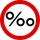A. Calculate 1.5 ‰ from 4.5 liters of blood. Express the result in milliliters. B. Calculate three ‰ from € 50,000. C. The tray they brought breakfast to the landlord's bed is made of an alloy that contains 830 ‰ of silver. How much silver did they use
• FighterA military fighter flies at an altitude of 10 km. From the ground position, it was aimed at an altitude angle of 23° and 12 seconds later at an altitude angle of 27°. Calculate the speed of the fighter in km/h.
• Surface and volumeFind the surface and volume of a cuboid whose dimensions are 1 m, 50 cm, and 6 dm.
• Copper Cu wireCopper wire with a diameter of 1 mm and a weight of 350 g is wound on a spool. Calculate its length if the copper density is p = 8.9 g/cm cubic.
• Central angleWhat is the length of the arc of a circle with a diameter of 46 cm, which belongs to a central angle of 30°?
• Cyclist 12What is the average speed of a cycle traveling at 20 km in 60 minutes in km/h?
• Observation towerFrom the observation tower at a height of 105 m above sea level, the ship is aimed at a depth angle of 1° 49´. How far is the ship from the base of the tower?